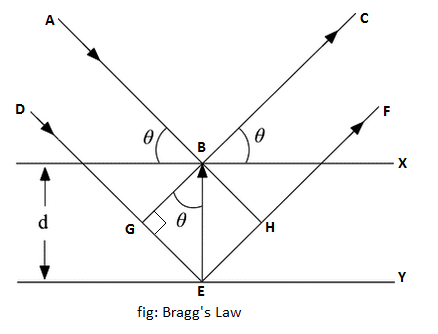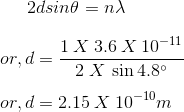# Bragg’s Law

Bragg’s law was first proposed by Sir William Bragg and his son Sir Lawrence Bragg. They studied about the diffraction of X-rays and devised a method for determining the wavelength of X-rays.

## Bragg’s Law Statement

When a monochromatic X-rays are incident upon a crystal, atoms in different layers acts as a source of scattering radiation of same wavelength. The intensity of the reflected beam will be maximum at certain incident angle when the path difference between two reflected wave from two different planes is an integral multiple of wavelength of X-rays.

```i.e For maximum intensity,
Path difference = nλ
where n = 1,2,3,... and λ = wavelength of X-rays```

Let us consider a parallel beam of monochromatic X-rays AB and DE of wavelength λ is incident on parallel crystal planes X and Y respectively, both with a equal glancing angle θ. Ray AB strikes an atom at B and is reflected along BC. Similarly, ray DE strikes an atom at E and is reflected along EF.

Now, draw BG and BH perpendicular to DE and EF respectively. From above figure, the path difference between the rays DEF and ABC is

path difference = GE + EH

```From triangle BGE and BEH,
EH = GE = BEsinθ
or, EH = GE = dsinθ
where d = interplaner spacing

So, path difference = 2dsinθ```

We know, for maximum intensity

```Path difference = nλ
or, 2dsinθ = nλ```

This is called Bragg’s Law.

Here n gives the order of diffraction.

In general,

`2dsinθn = nλ`

## Numerical Problem for Bragg’s Law

X-rays of wavelength 3.6 X 10-11m undergoes first order reflection at a glancing angle of 4.8° from a crystal. Find the spacing of the atomic planes in the crystal.

Solution:

Wavelength of X-ray(λ) = 3.6 X 10-11m

Glancing angle(θ) = 4.8°

Order of diffraction (n) = 1

Let the spacing of atomic planes in the crystal be d. Then using Bragg’s law, we haveHence the spacing of the atomic planes is 2.5 X 10-10m.